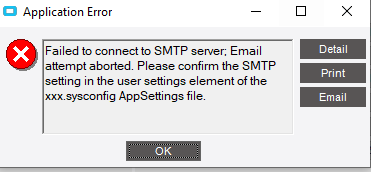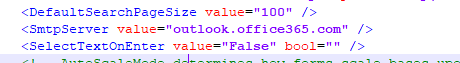# Send Email from Customization Code

Hi all!

I am making a Customization on the QuoteEntry module.
When the user press a button I need to do some things (that are done correctly) and at last send an email.
The problem is that every time Epicor tries to send the email it throws an errorI know for sure that if I set a BPM to send an email, it will send it without errors.

Am I missing some parameters in my code?

``````' CODE FOR CHECK QUOTE PRICE
oTrans.PushStatusText("Check Quote Price", true)
'EpimessageBox.Show("Start")
Dim args2 As EmailArgs = New EmailArgs()
dim UserEmail as string = ""
for each HedOrdeRow as datarow in OrderHed_Row.Dataview.Table.Rows
if HedOrdeRow.Item("Quote_Related_c") <> 0
'EpimessageBox.Show("Entro")
dim QuoteNum as integer = Convert.ToInt32(HedOrdeRow.Item("Quote_Related_c"))
dim OrderNum as integer = Convert.ToInt32(HedOrdeRow.Item("OrderNum"))
dim OrderTotalCharges as double = Convert.ToDouble(HedOrdeRow.Item("DocTotalCharges"))
dim OrderTotalMisc as double = Convert.ToDouble(HedOrdeRow.Item("DocTotalMisc"))
dim TotalOrder as double = OrderTotalCharges + OrderTotalMisc
dim QuoteTotalCharges as double
dim QuoteTotalMisc as double
dim TotalQuote as double
dim CurFolderSeq as integer = 0
'EpimessageBox.Show("Quote Related: " + QuoteNum.ToString() + " - OrderNum: " + OrderNum.ToString() + " TotalCh: " + OrderTotalCharges.ToString() + " Misc: " + OrderTotalMisc.ToString() + " Total: " + TotalOrder.ToString())
Try
Dim dqds As Ice.BO.DynamicQueryDataSet = DQAdapter.GetQueryDesignData("GetTotalPriceOfQuote")
Dim dqds1 As Ice.BO.QueryExecutionDataSet = DQAdapter.GetQueryExecutionParameters(dqds)
AssignQparameter(dqds1, "QuoteNum", QuoteNum)
Dim dsPart As System.Data.DataSet = DQAdapter.QueryResults
if dsPart.Tables(0).Rows.Count > 0 then
dim dtTable as DataTable = new DataTable()
dtTable = dsPart.Tables(0)
dim dv as DataView = dtTable.DefaultView
dim dtSorted as DataTable = new DataTable()
dtSorted = dv.ToTable()
QuoteTotalCharges = Convert.ToDouble(dtSorted.Rows(0)("QuoteHed_DocTotalGrossValue"))
QuoteTotalMisc = Convert.ToDouble(dtSorted.Rows(0)("QuoteHed_DocTotalMiscAmt"))
TotalQuote = QuoteTotalCharges + QuoteTotalMisc
'EpimessageBox.Show("Quote Total: " + QuoteTotalCharges.ToString() + " Misc: " + QuoteTotalMisc.ToString() + " Total: " + TotalQuote.ToString())
end if
Catch ex As System.Exception
ExceptionBox.Show(ex)
End Try
Try
Dim dqds As Ice.BO.DynamicQueryDataSet = DQAdapter.GetQueryDesignData("GetEmailEntryPerson")
Dim dqds1 As Ice.BO.QueryExecutionDataSet = DQAdapter.GetQueryExecutionParameters(dqds)
AssignQparameter(dqds1, "CurFolderSeq", CurFolderSeq)
Dim dsPart As System.Data.DataSet = DQAdapter.QueryResults
if dsPart.Tables(0).Rows.Count > 0 then
dim dtTable as DataTable = new DataTable()
dtTable = dsPart.Tables(0)
dim dv as DataView = dtTable.DefaultView
dim dtSorted as DataTable = new DataTable()
dtSorted = dv.ToTable()
'EpimessageBox.Show("Email: " + UserEmail.ToString())
end if
Catch ex As System.Exception
ExceptionBox.Show(ex)
End Try
If TotalQuote <> TotalOrder then
Epimessagebox.show("ATTENTION: " + vbCrLf + "The Order has a total of: " + TotalOrder.ToString() + " (Lines: " + OrderTotalCharges.ToString() + " , MiscCharges: " + OrderTotalMisc.ToString() + ")" + vbCrLf + "The Quote has a total of: " + TotalQuote.ToString() + " (Lines: " + QuoteTotalCharges.ToString() + " , MiscCharges: " + QuoteTotalMisc.ToString() + ")")
args2.Subject = "Order: " + OrderNum.ToString() + ". Different Price than the Quote related"
args2.MessageBody = "```Order: " + OrderNum.ToString() + vbCrLf + "Total Price of the Lines: " + OrderTotalCharges.ToString() + vbCrLf + "Total MiscCharges: " + OrderTotalMisc.ToString() +  vbCrLf + vbCrLf + "Quote Related: " + QuoteNum.ToString() +  vbCrLf + "Total Price of the Lines: " + QuoteTotalCharges.ToString() + vbCrLf + "Total MiscCharges: " + QuoteTotalMisc.ToString() + vbCrLf + vbCrLf + "ARE DIFFERENT, PLEASE REVIEW THEM." + "```"
EmailHandler.SendMail(args2)
end if
end if
next
oTrans.PushStatusText("", false)
``````

I tried to look in the SysConfigFile and I only found the ‘SmtpServer’ key, but without any other fields linked to it.(I tried to change it to the ‘outlook.office365.com’ that I use in the Company Maintenance, but it does not work).

Can someone help me plase?
Thanks!

Did you set the SMTP configuration in the Company Maintenance Screen?

Yeah everything is set.If I do a BPM that sends an email, it works

What kind of object is EmailHandler? Where’s the code where you create it?

I actually solved it.
I had to use another code (and harcoded the password for the sender email).
I used SmtpClient

That’s what I use, but it would have been neat to know how to send it through Epicor!